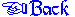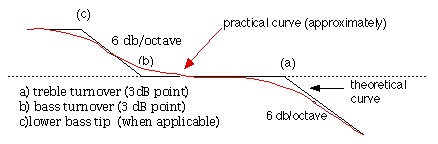##COMPUTER EQUALIZATION OF 78sTechnical Notes Index

Having had several enquiries about using a computer to make up the equalization curves I should say that on the whole I think the preferable method is to use a dedicated preamplifier: however in view of the cost the computer system may be acceptable, particularly if you don't have many records to transfer. I worked out some figures, and I have posted them below in case anyone wants to experiment.

The following figures assume a magnetic cartridge properly terminated (usually 47k for moving magnet/moving iron: moving coil as manufacturer's specs) into a flat response before filtering. Ceramic cartridges won't give you a useful result.

When you make up a curve, remember that the turnover point isn't a sharp 'knee' as in the theoretical curve, but the 3dB point where the slope and the flat sections intersect, as in this drawing:WESTREX is the easiest
Flat from 500 Hz upwards
+3dB at 250 Hz
+6 at 125
+12 at 62
+15 at 50
+18 at 30 and I should roll it off below that.

BLUMLEIN
Flat from 500 upwards
+3 at 250
+6 at 125
+12 at 50
+15 at 25 then roll it off below that

ffrr 78
+12 at 50
+3 at 150
0 at 300
0 at 1
-3 at 3.4kHz
-6 at 7 kHz
-9 at 10 kHz

DECCA 78
+11 at 50 Hz
+3 at 150 Hz
0 at 300 Hz
0 at 1.5kHz
-3 at 3.4 kHz
-6 at 7 kHz
-9 at 10 kHz

BSI 78
+17 at 25 Hz
+14 at 50Hz
+6 at 120
+3 at 350
0 at 700
0 at 1.5kHz
-3 at 3.2kHz
-6 at 6.5 kHz
-10 at 10 kHz.

## CONVERSION FROM RIAA

If you are using a preamplifier which equalizes a phono input for RIAA (LPs) the following figures will convert (approximately) to the two main 78 curves:

TO CONVERT FROM RIAA TO WESTREX
-2 at 500 Hz and flat below that
+3 at 2 kHz
+6 at 4 kHz
+13 at 10 kHz

TO CONVERT FROM RIAA TO BLUMLEIN
-5 at 50 Hz and flat below that
-3 at 250 Hz
+3 at 2 kHz
+6 at 4 kHz
+13 at 10 kHz

TO CONVERT FROM RIAA TO BSI 78
-3 at 50 Hz and flat below that
-1 at 350 Hz and 500 Hz
+1 at 2 kHz and 3 kHz
+3 at 10 kHz

Note that in some cases you need as much as a 37dB gain swing, which is a lot!

The figures are pretty close, one or two may be a dB or so out, but in practice most records only approximate the theoretical curve. Try to get a smooth 6dB/octave slope between the 6db and the next points.